# Sector Of A Circle

A circle has always been an important shape among all geometrical figures. There are various concepts and formulas related to a circle. The sectors and segments are perhaps most useful of them. In this article, we shall focus on the concept of a sector of a circle along with area and perimeter of a sector.

##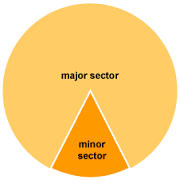A sector is said to be a part of a circle made of the arc of the circle along with its two radii. It is a portion of the circle formed by a portion of the circumference (arc) and radii of the circle at both endpoints of the arc. The shape of a sector of a circle can be compared with a slice of pizza or a pie. Before we start learning more about sector, first let us learn some basics of the circle.

What is a Circle?

A circle is a locus of points equidistant from a given point located at the centre of the circle. The common distance from the centre of the circle to its point is called radius. Thus, the circle is defined by its centre (o) and radius (r). A circle is also defined by two of its properties such as area and perimeter. The formulas for both the measures of the circle are given by;

 Area of a circle = πr2 Perimeter of a circle = 2πr

## What is Sector of a circle?

The sector is basically a portion of a circle which could be defined based on these three points mentioned below:

• A circular sector is the portion of a disk enclosed by two radii and an arc.
• A sector divides the circle into two regions namely Major and Minor Sector.
• The smaller area is known as the Minor Sector whereas the region having Greater area is known as Major Sector.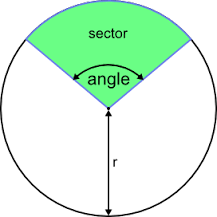## Area of a sector

In a circle with radius r and centre at O, let ∠POQ = θ (in degrees) be the angle of the sector. Then, the area of a sector of circle formula is calculated using the unitary method.

For the given angle the Area of a sector is represented by:

Angle of the sector is 360°, area of the sector i.e. the Whole circle = πr2

When the Angle is 1°, area of sector = πr2/360°

So, when the angle is θ, area of sector, OPAQ,  is defined as;

 A = θ/360° ×  πr2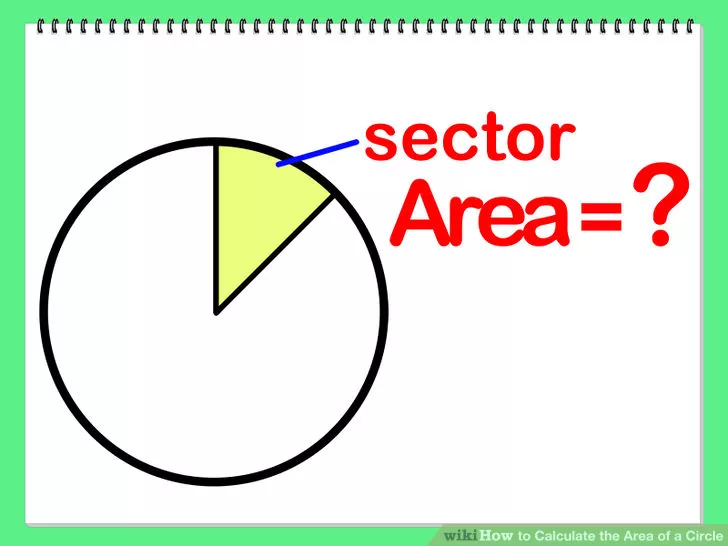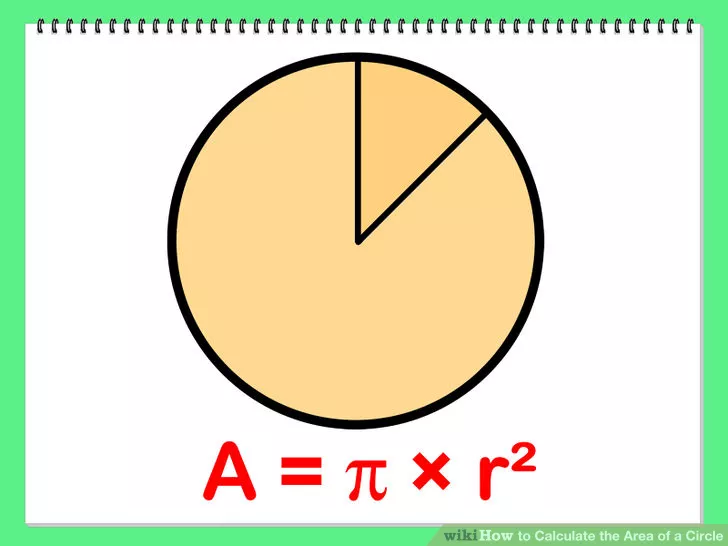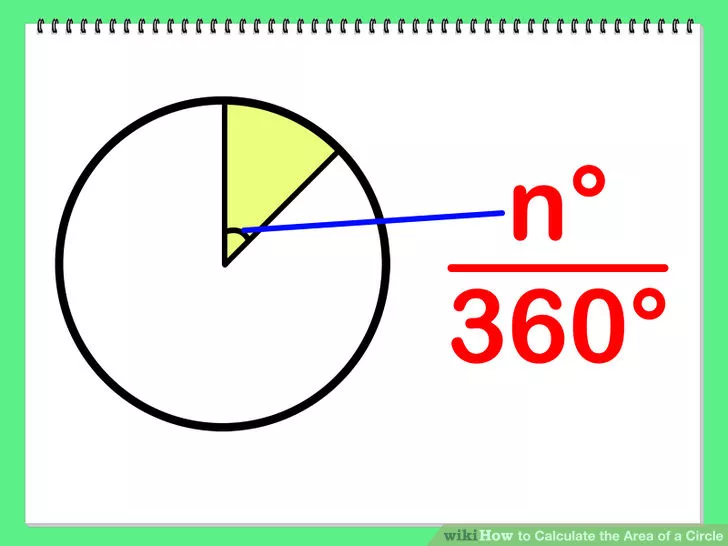Let the angle be 45 °, therefore the circle will be divided into 8 parts, as per the given in the below figure;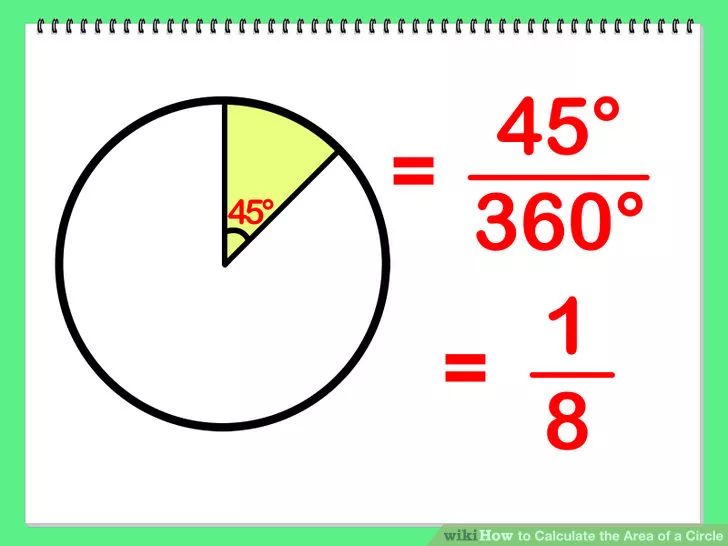Now the area of the sector for the above figure can be calculated as ⅛(3.14×r×r).

Thus the Area of a sector is calculated as:

 A = θ/360  × 22/7 × r2

### Length of the Arc of Sector Formula

Similarly, the length of the arc (PQ) of the sector with angle θ, is given by;

 l = θ/360  × 2πr

Area of Sector with respect to Length of the Arc

If the length of the arc of the sector is given instead of the angle of the sector, there is a different way to calculate the area of the sector. Let the length of the arc be l. For the radius of a circle equal to r units, an arc of length r units will subtend 1 radian at the centre. It can be hence concluded that an arc of length l will subtend l/r, the angle at the centre. So, if l is the length of the arc, r is the radius of the circle and θ is the angle subtended at the centre, then;

θ = l/r, where θ is in radians.

When the angle of the sector is 2π, area of the sector i.e. the whole circle = πr2

When the angle is 1, the area of the sector = πr2/2π = r2/2

So, when the angle is θ, area of the sector = θ ×  r2/2

A = l/r × r2/2

 A = lr/2

Some examples for better understanding are discussed from here on.

### Examples

Example 1: If the angle of the sector with radius 4 units is 45°, then find the length of the sector.

Solution: Area = θ/360° ×  πr2

= 45°/360° × 22/7 × 4 × 4

= 44/7 square units

The length of the same sector = θ/360° × 2πr

l = 45°/360° × 2 × 22/7 × 4

l = 22/7

Example 2: Find the area of the sector when the radius of the circle is 16 units and the length of the arc is 5 units.

Solution: If the length of the arc of a circle with radius 16 units is 5 units, the area of the sector corresponding to that arc is;

A = lr/2 = (5 × 16)/2 = 40 square units.

## Perimeter of a Sector

The perimeter of the sector of a circle is the length of two radii along with the arc that makes the sector. In the following diagram, a sector is shown in white colour.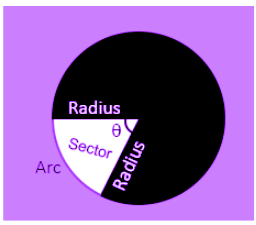The perimeter should  be calculated by doubling the radius and then adding it to the length of the arc

### Perimeter of a Sector Formula

The formula for the perimeter of the sector of a circle is given below :

Perimeter of sector = 2 radius + arc length

Arc length is calculated using the relation :

Arc length = radius × central angle

Therefore,

 Perimeter of a Sector = 2 Radius + (radius × central angle)

### Example

A circular arc whose radius is 12 cm, makes an angle of 30° at the centre. Find the perimeter of the sector formed. Use π = 3.14.

Solution : Given that r = 12 cm,

θ = 30° = 30° × (π/180°) = π/6

Perimeter of sector is given by the formula;

P = 2 r + r θ

P = 2 . 12 + 12 . π/6

P = 24 + 2 π

P = 24 + 6.28 = 30.28

Hence, Perimeter of sector is 30.28 cm